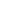The glossary is a collection of terms, definitions and expressions used in the debt capital markets. It has been sorted alphabetically by term. Select a page:   A-B   C   D-E   F-I   J-M   N-Q   R-S   T-ZYieldCurve.com glossary terms containing "yield curve" Par yield curve: A curve plotting maturity against yield for bonds priced at par. Yield curve: Graphical representation of the maturity structure of interest rates, plotting yields of bonds that are all of the same class or credit quality against the maturity of the bonds.YieldCurve.com glossary definitions containing "yield curve" Asset & Liability Management (ALM): The practice of matching the term structure and cash flows of an organisation's asset and liability portfolios to maximise returns and minimise risk. Also includes the deliberate mis-matching of cash flows to take account of views on the short-term yield curve. Benchmark: A bond whose terms set a standard for the market. The benchmark usually has the greatest liquidity, the highest turnover and is usually the most frequently quoted. It also usually trades expensive to the yield curve, due to higher demand for it amongst institutional investors. Convexity: A measure of the curvature of a bond's price/yield curve (mathematically). Yield-curve option: Option that allows purchasers to take a view on a yield curve without having to take a view about a market's direction. Yield-curve swap: Swap in which the index rates of the two interest streams are at different points on the yield curve. Both payments are refixed with the same frequency whatever the index rate. Zero-coupon: A zero-coupon security is one that does not pay a coupon. Its price is correspondingly less to compensate for this. A zero-coupon yield is the yield which a zero-coupon investment for that term would have if it were consistent with the par yield curve. Z-spread: The z-spread is a spread of a bond yield to a yield curve. It is the basis point spread that would need to be added to the implied spot yield curve such that the discounted cashflows of a bond are equal to its present value (its current market price). Each bond cashflow would be discounted by the relevant spot rate for its maturity term. This differs from a conventional swap spread which has been calculated using the bond's yield-to-maturity to discount all its cashflows. Both spreads can be viewed as the coupon of a swap market annuity of equivalent credit risk of the bond being valued.

# GlossaryThe YieldCurve.com glossary is a list of terms commonly encountered in the debt capital markets.
 The glossary is a collection of terms, definitions and expressions used in the debt capital markets. It has been sorted alphabetically by term. Select a page:   A-B   C   D-E   F-I   J-M   N-Q   R-S   T-ZHome   What's New   Market Research   Presentations
RATE yield curve model   Excel Spreadsheets
© YieldCurve.comDisclaimerweb design